R Markdown

This is an R Markdown document. For more details on using R Markdown see http://rmarkdown.rstudio.com.

When you click the Knit button a document will be generated that includes both content as well as the output of any embedded R code chunks within the document.

The syntax on line 19 then the subsequent on line 56 tells R Markdown to run R code without the asterisks embeds the subsequent code as embedded inside the R Markdown file

library(dplyr)
##
## Attaching package: 'dplyr'
## The following objects are masked from 'package:stats':
##
##     filter, lag
## The following objects are masked from 'package:base':
##
##     intersect, setdiff, setequal, union
library(plyr)
## ------------------------------------------------------------------------------
## You have loaded plyr after dplyr - this is likely to cause problems.
## If you need functions from both plyr and dplyr, please load plyr first, then dplyr:
## library(plyr); library(dplyr)
## ------------------------------------------------------------------------------
##
## Attaching package: 'plyr'
## The following objects are masked from 'package:dplyr':
##
##     arrange, count, desc, failwith, id, mutate, rename, summarise,
##     summarize
library(doBy)
library(tidyr)
library(glmnet)
##
## Attaching package: 'Matrix'
## The following objects are masked from 'package:tidyr':
##
##     expand, pack, unpack
library(ggplot2)

library(randomForest)
## randomForest 4.6-14
## Type rfNews() to see new features/changes/bug fixes.
##
## Attaching package: 'randomForest'
## The following object is masked from 'package:ggplot2':
##
##     margin
## The following object is masked from 'package:dplyr':
##
##     combine
setwd("C:/Users/jlariv/OneDrive/Econ 404/")
oj <- mydata
colnames(mydata)[1-17]
##   "store"    "brand"    "week"     "logmove"  "feat"     "price"
##   "AGE60"    "EDUC"     "ETHNIC"   "INCOME"   "HHLARGE"  "WORKWOM"
##  "HVAL150"  "SSTRDIST" "SSTRVOL"  "CPWVOL5"
#reduce decimal places
is.num=sapply(mydata, is.numeric)
mydata[is.num] = lapply(mydata[is.num], round, 4)

#CREATE LAGGED DATA with recycled code
#First why to add in lagged weeks
df1 <-oj
df1$week<-df1$week+1  # df1 now has NEXT week and not the current one.  If we merge this by weeks now, this is last week's price (e.g., "lagged price").
df2 <-oj
df2$week<-df1$week+2

myvars <- c("price", "logmove","week", "brand","store", "feat")
df1 <- df1[myvars]
names(df1)[names(df1)=="price"] = "price_t1"
names(df1)[names(df1)=="feat"] = "feat_t1"
names(df1)[names(df1)=="logmove"] = "logmove_t1"
lagged <- merge(oj, df1, by=c("brand","store","week"))
#NOTE: The number of observations decreased.  Why?  You've just lost (at least) one week's worth of data at each store.
lagged=lagged[order(lagged$week,lagged$store),]
lagged=lagged[order(lagged$store,lagged$week),]
#Comparing this to above, Store two is nowhere to be found.  There was missing data for consecutive weeks.  As a result, it gets dropped.

#Now do the same this with two weeks of lagged data.

df2 <- df2[myvars]
names(df2)[names(df2)=="price"] = "price_t2"
names(df2)[names(df2)=="feat"] = "feat_t2"
names(df2)[names(df2)=="logmove"] = "logmove_t2"
lagged_RF <- merge(lagged, df2, by=c("brand","store","week"))

#Repeat entire process with additional lag
df3 <- oj
df2$week<-df1$week+2
df3 <- df3[myvars]
names(df3)[names(df3)=="price"] = "price_t3"
names(df3)[names(df3)=="feat"] = "feat_t3"
names(df3)[names(df3)=="logmove"] = "logmove_t3"
lagged_RF <- merge(lagged_RF, df3, by=c("brand","store","week"))

#drop unknown features to avoid spliting on these features.
lagged_RF$SSTRDIST <- NULL lagged_RF$SSTRVOL <- NULL
lagged_RF$CPWVOL5 <- NULL lagged_RF$CPDIST5 <- NULL

# NOTE: This code does not scale in the number of products; for that we need to utilize loops or some prepackaged function.
lagged_RF_D <- subset(lagged_RF,brand=="dominicks")
lagged_RF_M <- subset(lagged_RF,brand=="minute.maid")
lagged_RF_T <- subset(lagged_RF,brand=="tropicana")

#Dominicks rename
names(lagged_RF_D)[names(lagged_RF_D)=="price_t3"] = "price_t3_D"
names(lagged_RF_D)[names(lagged_RF_D)=="price_t2"] = "price_t2_D"
names(lagged_RF_D)[names(lagged_RF_D)=="price_t1"] = "price_t1_D"
names(lagged_RF_D)[names(lagged_RF_D)=="price"] = "price_D"

names(lagged_RF_D)[names(lagged_RF_D)=="feat_t3"] = "feat_t3_D"
names(lagged_RF_D)[names(lagged_RF_D)=="feat_t2"] = "feat_t2_D"
names(lagged_RF_D)[names(lagged_RF_D)=="feat_t1"] = "feat_t1_D"
names(lagged_RF_D)[names(lagged_RF_D)=="feat"] = "feat_D"

names(lagged_RF_D)[names(lagged_RF_D)=="logmove_t3"] = "logmove_t3_D"
names(lagged_RF_D)[names(lagged_RF_D)=="logmove_t2"] = "logmove_t2_D"
names(lagged_RF_D)[names(lagged_RF_D)=="logmove_t1"] = "logmove_t1_D"
names(lagged_RF_D)[names(lagged_RF_D)=="logmove"] = "logmove_D"

#MM rename
names(lagged_RF_M)[names(lagged_RF_M)=="price_t3"] = "price_t3_M"
names(lagged_RF_M)[names(lagged_RF_M)=="price_t2"] = "price_t2_M"
names(lagged_RF_M)[names(lagged_RF_M)=="price_t1"] = "price_t1_M"
names(lagged_RF_M)[names(lagged_RF_M)=="price"] = "price_M"

names(lagged_RF_M)[names(lagged_RF_M)=="feat_t3"] = "feat_t3_M"
names(lagged_RF_M)[names(lagged_RF_M)=="feat_t2"] = "feat_t2_M"
names(lagged_RF_M)[names(lagged_RF_M)=="feat_t1"] = "feat_t1_M"
names(lagged_RF_M)[names(lagged_RF_M)=="feat"] = "feat_M"

names(lagged_RF_M)[names(lagged_RF_M)=="logmove_t3"] = "logmove_t3_M"
names(lagged_RF_M)[names(lagged_RF_M)=="logmove_t2"] = "logmove_t2_M"
names(lagged_RF_M)[names(lagged_RF_M)=="logmove_t1"] = "logmove_t1_M"
names(lagged_RF_M)[names(lagged_RF_M)=="logmove"] = "logmove_M"

#Trop rename
names(lagged_RF_T)[names(lagged_RF_T)=="price_t3"] = "price_t3_T"
names(lagged_RF_T)[names(lagged_RF_T)=="price_t2"] = "price_t2_T"
names(lagged_RF_T)[names(lagged_RF_T)=="price_t1"] = "price_t1_T"
names(lagged_RF_T)[names(lagged_RF_T)=="price"] = "price_T"

names(lagged_RF_T)[names(lagged_RF_T)=="feat_t3"] = "feat_t3_T"
names(lagged_RF_T)[names(lagged_RF_T)=="feat_t2"] = "feat_t2_T"
names(lagged_RF_T)[names(lagged_RF_T)=="feat_t1"] = "feat_t1_T"
names(lagged_RF_T)[names(lagged_RF_T)=="feat"] = "feat_T"

names(lagged_RF_T)[names(lagged_RF_T)=="logmove_t3"] = "logmove_t3_T"
names(lagged_RF_T)[names(lagged_RF_T)=="logmove_t2"] = "logmove_t2_T"
names(lagged_RF_T)[names(lagged_RF_T)=="logmove_t1"] = "logmove_t1_T"
names(lagged_RF_T)[names(lagged_RF_T)=="logmove"] = "logmove_T"

#temp DFs for merging to other brands' sales
#Trop for D and M
myvars_T <- c("feat_t3_T", "feat_t2_T", "feat_t1_T","feat_T", "price_t3_T", "price_t2_T", "price_t1_T","price_T", "logmove_T","logmove_t1_T", "logmove_t2_T", "logmove_t3_T","store","week")
temp_T <- lagged_RF_T[myvars_T]

#Dom for T and M
myvars_D <- c("feat_t3_D", "feat_t2_D", "feat_t1_D","feat_D", "price_t3_D", "price_t2_D", "price_t1_D","price_D","logmove_D","logmove_t1_D", "logmove_t2_D", "logmove_t3_D","store","week")
temp_D <- lagged_RF_D[myvars_D]

#MM for T and D
myvars_M <- c("feat_t3_M", "feat_t2_M", "feat_t1_M","feat_M", "price_t3_M", "price_t2_M", "price_t1_M","price_M","logmove_M","logmove_t1_M", "logmove_t2_M", "logmove_t3_M","store","week")
temp_M <- lagged_RF_M[myvars_M]

lagged_RF_D <- merge(lagged_RF_D, temp_T, by=c("store","week"))
lagged_RF_D <- merge(lagged_RF_D, temp_M, by=c("store","week"))

lagged_RF_M <- merge(lagged_RF_M, temp_T, by=c("store","week"))
lagged_RF_M <- merge(lagged_RF_M, temp_D, by=c("store","week"))

lagged_RF_T <- merge(lagged_RF_T, temp_D, by=c("store","week"))
lagged_RF_T <- merge(lagged_RF_T, temp_M, by=c("store","week"))

#Train the model excluding contemporaneous features
## DOMINICKS
DQ <- lagged_RF_D
DQ$feat_D <- NULL DQ$price_D <- NULL
DQ$feat_M <- NULL DQ$price_M <- NULL
DQ$feat_T <- NULL DQ$price_T <- NULL
range(DQ$week) ##  43 160 #Make sure to remove store variables since they are numeric and likely uninformative as such. The store FEs will be picked up by sociodemographics. Adjusting week to be a linear time trend. DQ$week <-DQ$week-42 colnames(DQ) ##  "store" "week" "brand" "logmove_D" "AGE60" ##  "EDUC" "ETHNIC" "INCOME" "HHLARGE" "WORKWOM" ##  "HVAL150" "price_t1_D" "logmove_t1_D" "feat_t1_D" "price_t2_D" ##  "logmove_t2_D" "feat_t2_D" "price_t3_D" "logmove_t3_D" "feat_t3_D" ##  "feat_t3_T" "feat_t2_T" "feat_t1_T" "price_t3_T" "price_t2_T" ##  "price_t1_T" "logmove_T" "logmove_t1_T" "logmove_t2_T" "logmove_t3_T" ##  "feat_t3_M" "feat_t2_M" "feat_t1_M" "price_t3_M" "price_t2_M" ##  "price_t1_M" "logmove_M" "logmove_t1_M" "logmove_t2_M" "logmove_t3_M" DQ.rf <- randomForest(logmove_D ~ AGE60+EDUC+ETHNIC+INCOME+HHLARGE+WORKWOM+HVAL150+price_t1_D+feat_t1_D+price_t2_D+feat_t2_D+price_t3_D+feat_t3_D+feat_t3_T+feat_t2_T+feat_t1_T+price_t3_T+price_t2_T+price_t1_T+feat_t3_M+feat_t2_M+feat_t1_M+price_t3_M+price_t2_M+price_t1_M++logmove_t1_M+logmove_t2_M+logmove_t3_M+logmove_t1_D+logmove_t2_D+logmove_t3_D+logmove_t1_T+logmove_t2_T+logmove_t3_T, data = DQ, ntree = 100, keep.forest = TRUE) DQ$pred_logmove_D = predict(DQ.rf)
DQ$resid_QD <- DQ$logmove_D- DQ$pred_logmove_D mean(DQ$resid_QD)
##  -0.0009238355
fig_DQ<-ggplot(DQ, aes(pred_logmove_D,logmove_D))+geom_point() + geom_smooth(method='lm') + geom_abline(intercept = 0, slope =1)

fig_DQ
## geom_smooth() using formula 'y ~ x'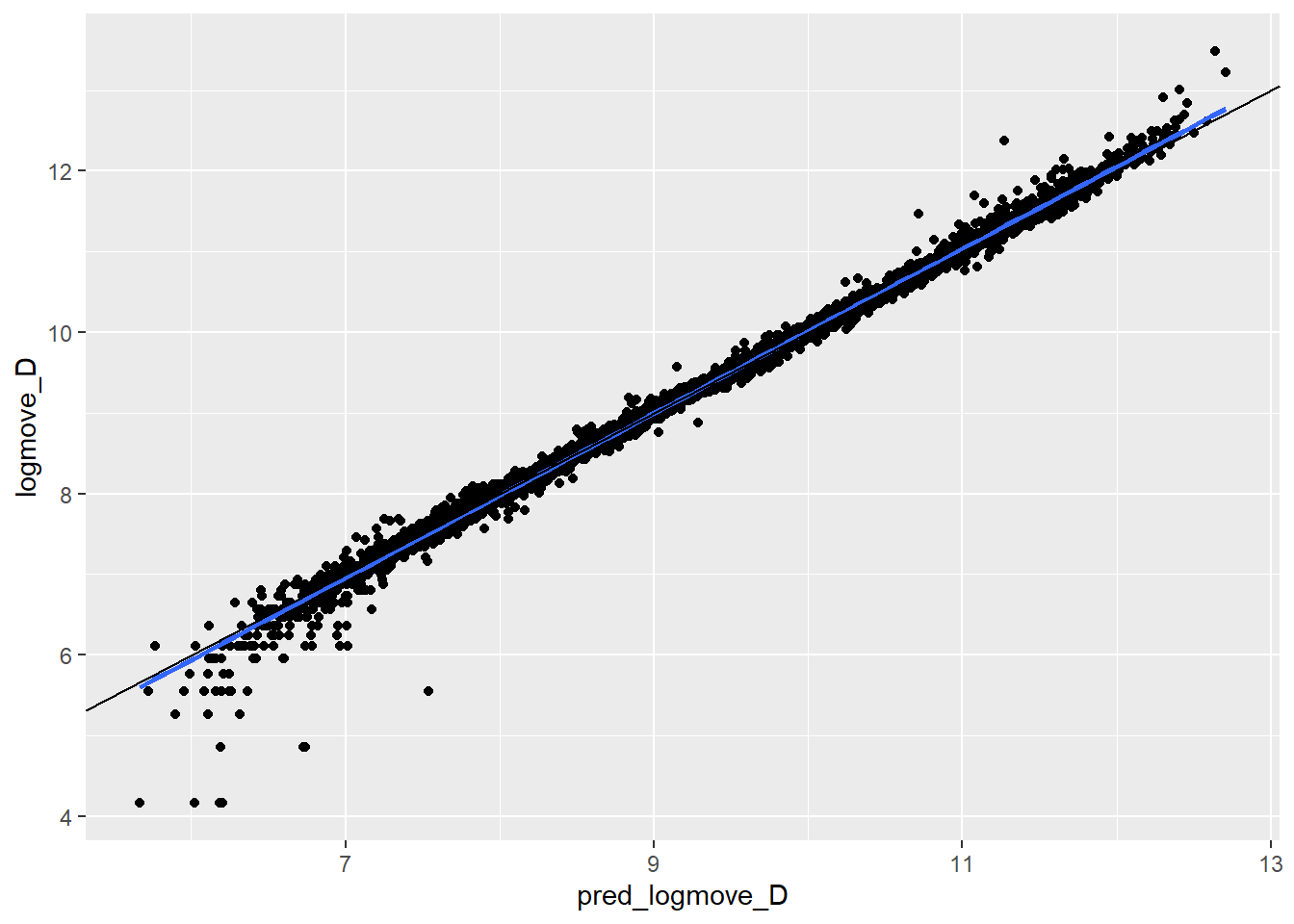DQ$resid_QD_2 <- (DQ$logmove_D- DQ$pred_logmove_D)^2 mean(DQ$resid_QD_2)
##  0.008887415
# mean is very close to zero.  Likely non-zero due to non-normal distribution of sales.

DP <- lagged_RF_D
DP$feat_D <- NULL DP$feat_M <- NULL
DP$price_M <- NULL DP$feat_T <- NULL
DP$price_T <- NULL DP$week <-DP$week-42 DP.rf <- randomForest(log(price_D) ~AGE60+EDUC+ETHNIC+INCOME+HHLARGE+WORKWOM+ HVAL150+price_t1_D+feat_t1_D+price_t2_D+feat_t2_D+price_t3_D+feat_t3_D+feat_t3_T+feat_t2_T+feat_t1_T+price_t3_T+price_t2_T+price_t1_T+feat_t3_M+feat_t2_M+feat_t1_M+price_t3_M+price_t2_M+price_t1_M+logmove_t1_M+logmove_t2_M+logmove_t3_M+logmove_t1_D+logmove_t2_D+logmove_t3_D+logmove_t1_T+logmove_t2_T+logmove_t3_T, data = DP, ntree = 100, keep.forest = TRUE) DP$pred_logprice_D = predict(DP.rf)
DP$resid_logP_D <- log(DP$price_D)- DP$pred_logprice_D mean(DP$resid_logP_D)
##  5.94934e-05
fig_DP<-ggplot(DP, aes(pred_logprice_D,log(price_D)))+geom_point() + geom_smooth(method='lm') + geom_abline(intercept = 0, slope =1)
fig_DP
## geom_smooth() using formula 'y ~ x'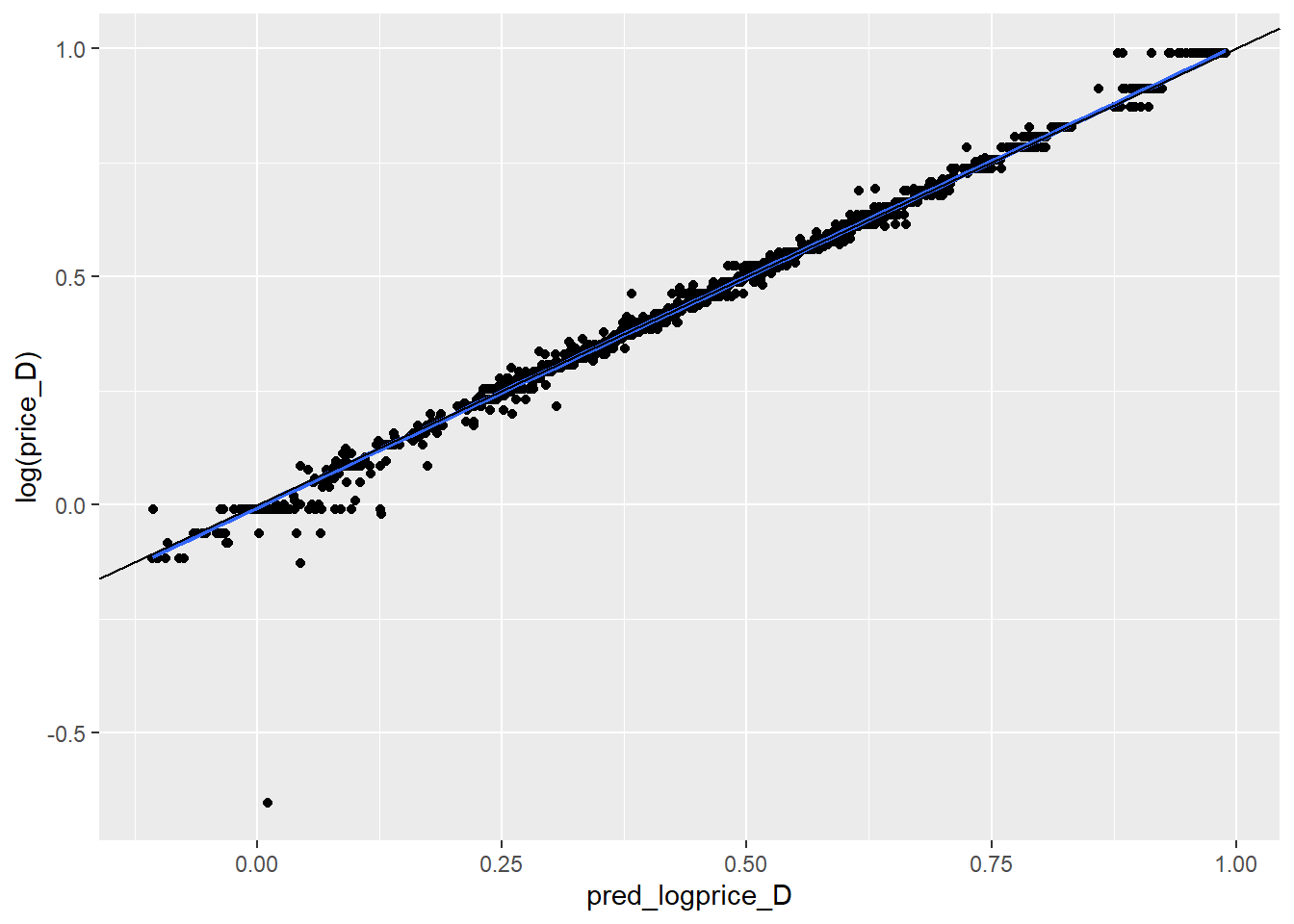DP$resid_logP_D_2 <- (log(DP$price_D)- DP$pred_logprice_D)^2 mean(DP$resid_logP_D_2)
##  0.000115961
# mean is very close to zero.

## MM
MQ <- lagged_RF_M
MQ$feat_D <- NULL MQ$price_D <- NULL
MQ$feat_M <- NULL MQ$price_M <- NULL
MQ$feat_T <- NULL MQ$price_T <- NULL
MQ$week <-MQ$week-42
MQ.rf <- randomForest(logmove_M ~ AGE60+EDUC+ETHNIC+INCOME+HHLARGE+WORKWOM+   HVAL150+price_t1_D+feat_t1_D+price_t2_D+feat_t2_D+price_t3_D+feat_t3_D+feat_t3_T+feat_t2_T+feat_t1_T+price_t3_T+price_t2_T+price_t1_T+feat_t3_M+feat_t2_M+feat_t1_M+price_t3_M+price_t2_M+price_t1_M+logmove_t1_M+logmove_t2_M+logmove_t3_M+logmove_t1_D+logmove_t2_D+logmove_t3_D+logmove_t1_T+logmove_t2_T+logmove_t3_T, data = MQ, ntree = 100, keep.forest = TRUE)
MQ$pred_logmove_M = predict(MQ.rf) MQ$resid_MQ <- MQ$logmove_M- MQ$pred_logmove_M
mean(MQ$resid_MQ) ##  -0.0009367125 fig_MQ<-ggplot(MQ, aes(pred_logmove_M,logmove_M))+geom_point() + geom_smooth(method='lm') + geom_abline(intercept = 0, slope =1) fig_MQ ## geom_smooth() using formula 'y ~ x'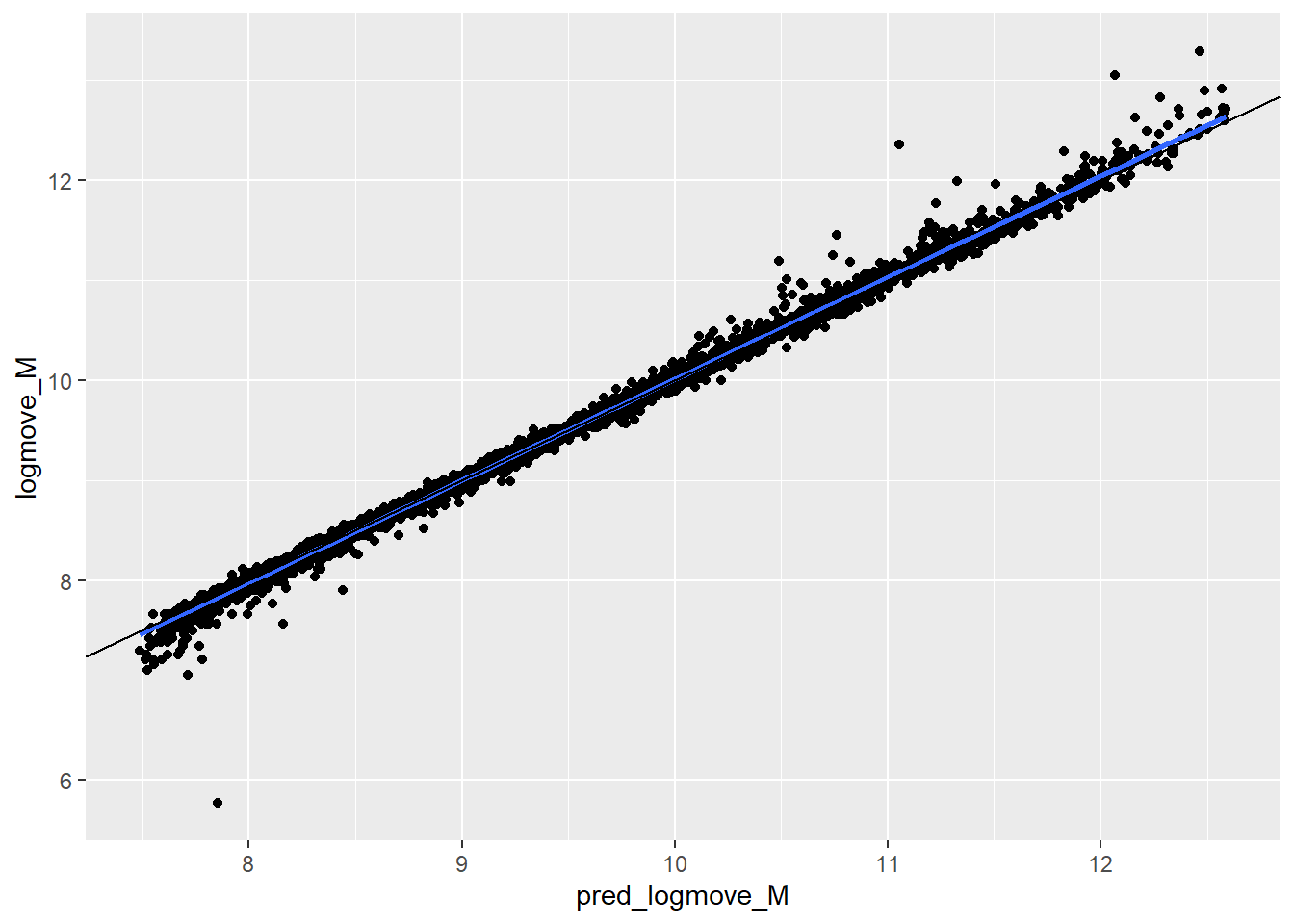MQ$resid_MQ_2 <- (MQ$logmove_M- MQ$pred_logmove_M)^2
mean(MQ$resid_MQ_2) ##  0.003872358 # mean is very close to zero. Likely non-zero due to non-normal distribution of sales. MP <- lagged_RF_M MP$feat_D <- NULL
MP$price_D <- NULL MP$feat_M <- NULL

MP$feat_T <- NULL MP$price_T <- NULL
MP$week <-MP$week-42
MP.rf <- randomForest(log(price_M) ~ AGE60+EDUC+ETHNIC+INCOME+HHLARGE+WORKWOM+ HVAL150+price_t1_D+feat_t1_D+price_t2_D+feat_t2_D+price_t3_D+feat_t3_D+feat_t3_T+feat_t2_T+feat_t1_T+price_t3_T+price_t2_T+price_t1_T+feat_t3_M+feat_t2_M+feat_t1_M+price_t3_M+price_t2_M+price_t1_M+logmove_t1_M+logmove_t2_M+logmove_t3_M+logmove_t1_D+logmove_t2_D+logmove_t3_D+logmove_t1_T+logmove_t2_T+logmove_t3_T, data = MP, ntree = 100, keep.forest = TRUE)
MP$pred_logprice_M = predict(MP.rf) MP$resid_logP_M <- log(MP$price_M)- MP$pred_logprice_M
mean(MP$resid_logP_M) ##  -2.495385e-05 fig_MP<-ggplot(MP, aes(pred_logprice_M,log(price_M)))+geom_point() + geom_smooth(method='lm') + geom_abline(intercept = 0, slope =1) fig_MP ## geom_smooth() using formula 'y ~ x'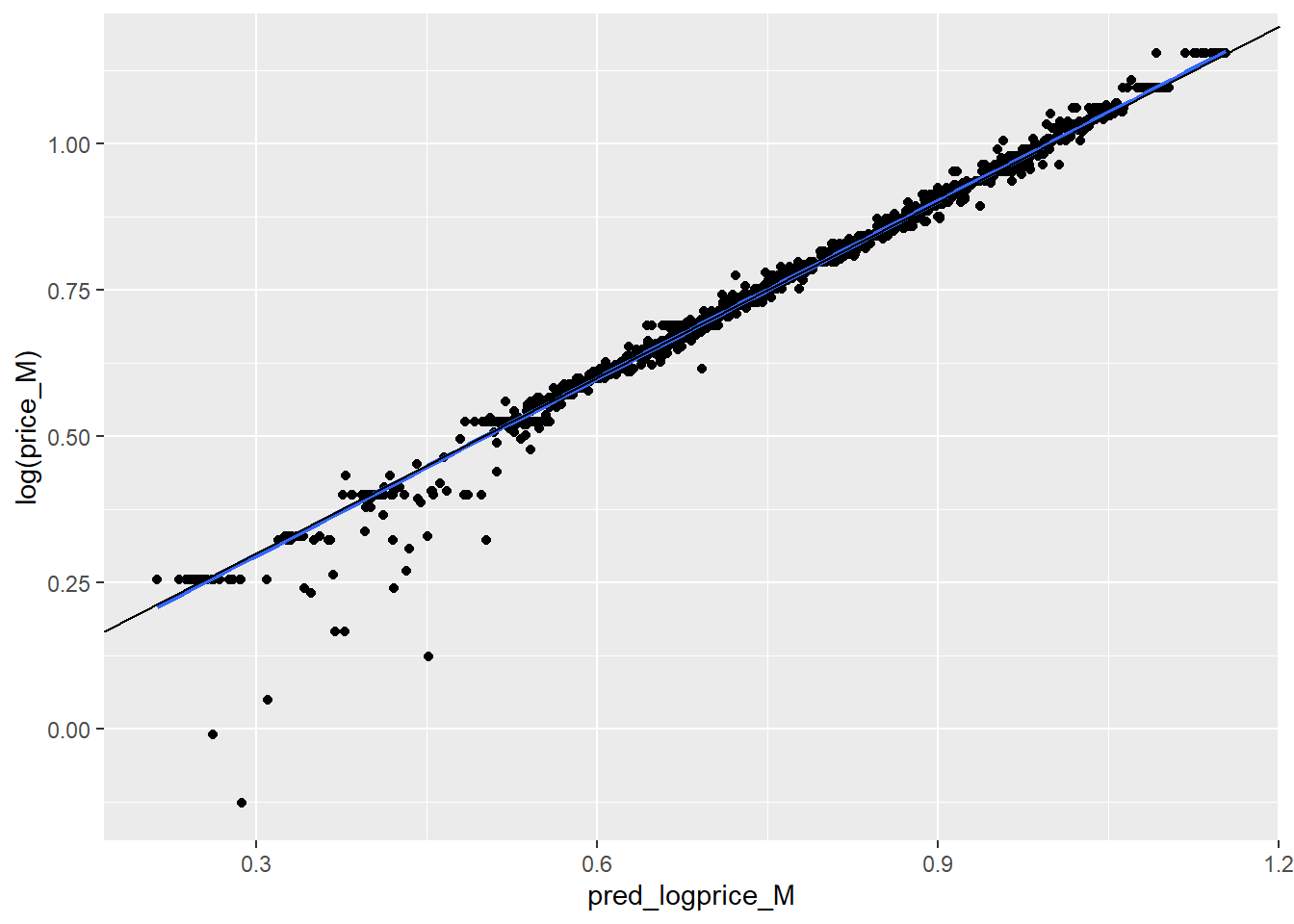MP$resid_logP_M_2 <- (log(MP$price_M)- MP$pred_logprice_M)^2
mean(MP$resid_logP_M_2) ##  0.0001100215 # mean is very close to zero. ## Tropicana TQ <- lagged_RF_T TQ$feat_D <- NULL
TQ$price_D <- NULL TQ$feat_M <- NULL
TQ$price_M <- NULL TQ$feat_T <- NULL
TQ$price_T <- NULL TQ$week <-TQ$week-42 TQ.rf <- randomForest(logmove_T ~ AGE60+EDUC+ETHNIC+INCOME+HHLARGE+WORKWOM+HVAL150+price_t1_D+feat_t1_D+price_t2_D+feat_t2_D+price_t3_D+feat_t3_D+feat_t3_T+feat_t2_T+feat_t1_T+price_t3_T+price_t2_T+price_t1_T+feat_t3_M+feat_t2_M+feat_t1_M+price_t3_M+price_t2_M+price_t1_M+logmove_t1_M+logmove_t2_M+logmove_t3_M+logmove_t1_D+logmove_t2_D+logmove_t3_D+logmove_t1_T+logmove_t2_T+logmove_t3_T, data = TQ, ntree = 100, keep.forest = TRUE) TQ$pred_logmove_T = predict(TQ.rf)
TQ$resid_TQ <- TQ$logmove_T- TQ$pred_logmove_T mean(TQ$resid_TQ)
##  -0.001301985
fig_TQ<-ggplot(TQ, aes(pred_logmove_T,logmove_T))+geom_point() + geom_smooth(method='lm') + geom_abline(intercept = 0, slope =1)
fig_TQ
## geom_smooth() using formula 'y ~ x'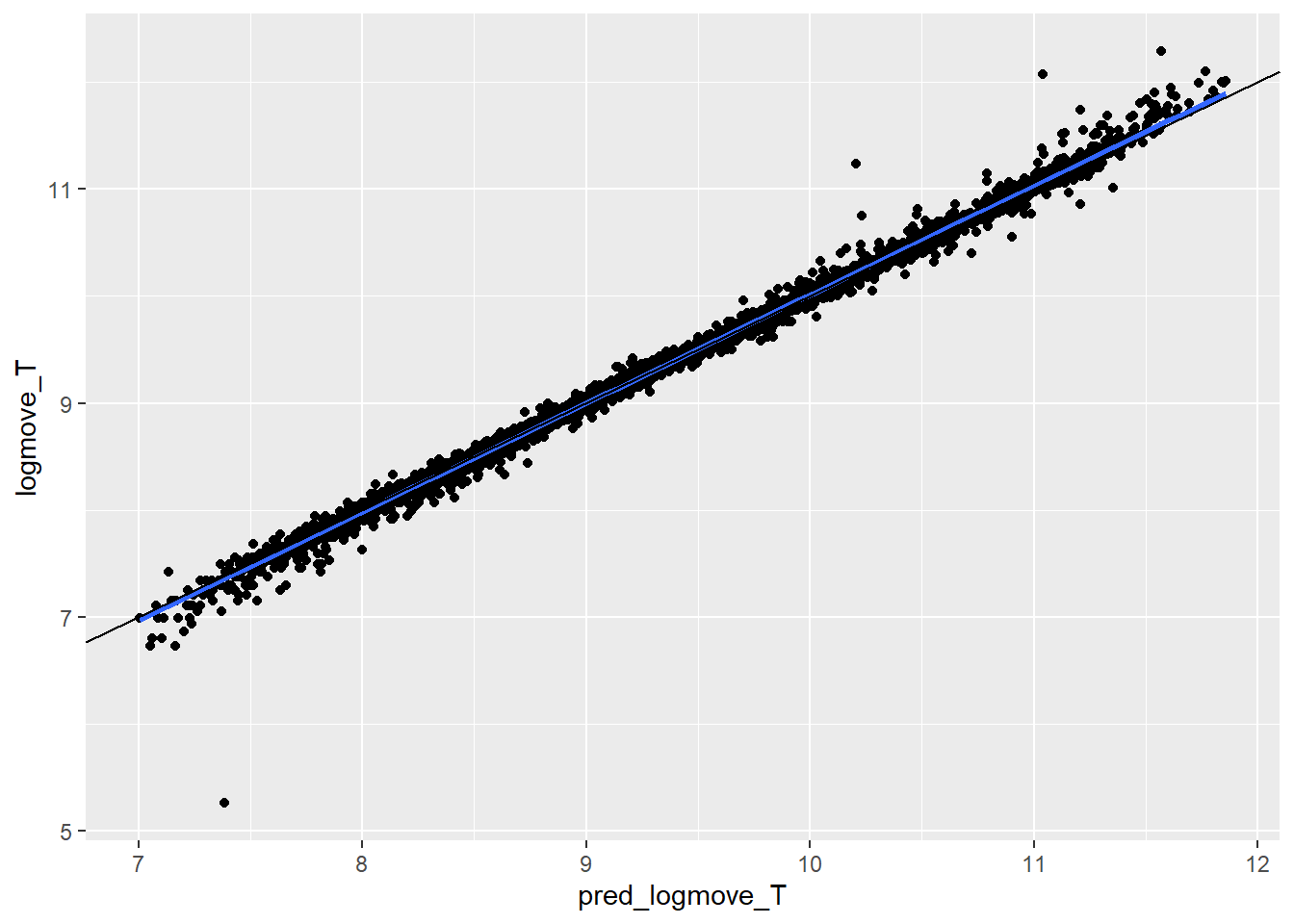TQ$resid_TQ_2 <- (TQ$logmove_T- TQ$pred_logmove_T)^2 mean(TQ$resid_TQ_2)
##  0.00334052
# mean is very close to zero.  Likely non-zero due to non-normal distribution of sales.

TP <- lagged_RF_T
TP$feat_D <- NULL TP$price_D <- NULL
TP$feat_M <- NULL TP$price_M <- NULL
TP$feat_T <- NULL TP$week <-TP$week-42 TP.rf <- randomForest(log(price_T) ~AGE60+EDUC+ETHNIC+INCOME+HHLARGE+WORKWOM+HVAL150+price_t1_D+feat_t1_D+price_t2_D+feat_t2_D+price_t3_D+feat_t3_D+feat_t3_T+feat_t2_T+feat_t1_T+price_t3_T+price_t2_T+price_t1_T+feat_t3_M+feat_t2_M+feat_t1_M+price_t3_M+price_t2_M+price_t1_M+logmove_t1_M+logmove_t2_M+logmove_t3_M+logmove_t1_D+logmove_t2_D+logmove_t3_D+logmove_t1_T+logmove_t2_T+logmove_t3_T, data = TP, ntree = 100, keep.forest = TRUE) TP$pred_logprice_T = predict(TP.rf)
TP$resid_logP_T <- log(TP$price_T)- TP$pred_logprice_T mean(TP$resid_logP_T)
##  -2.700007e-05
fig_TP<-ggplot(TP, aes(pred_logprice_T,log(price_T)))+geom_point() + geom_smooth(method='lm') + geom_abline(intercept = 0, slope =1)
fig_TP
## geom_smooth() using formula 'y ~ x'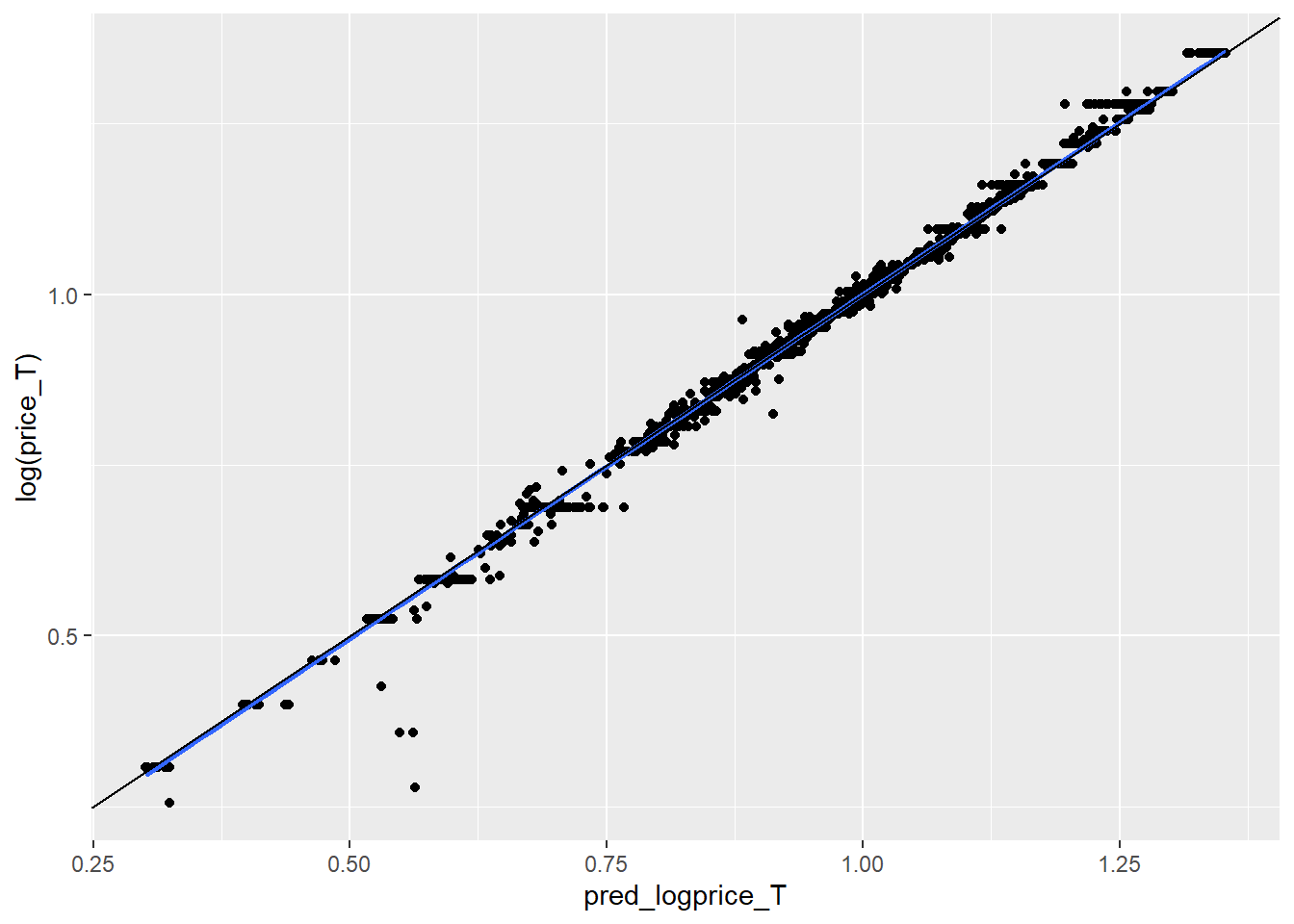TP$resid_logP_T_2 <- (log(TP$price_T)- TP$pred_logprice_T)^2 mean(TP$resid_logP_T_2)
##  5.44124e-05
# mean is very close to zero.

myvars_M <- c("feat_t3_M", "feat_t2_M", "feat_t1_M","feat_M", "price_t3_M", "price_t2_M", "price_t1_M","price_M","store","week")
temp_M <- lagged_RF_M[myvars_M]

temp_DP <- DP[c("store","week", "resid_logP_D")]
temp_MP <- MP[c("store","week", "resid_logP_M")]
temp_TP <- TP[c("store","week", "resid_logP_T")]

TQ <- merge(TQ, temp_DP, by=c("store","week"))
TQ <- merge(TQ, temp_MP, by=c("store","week"))
TQ <- merge(TQ, temp_TP, by=c("store","week"))

MQ <- merge(MQ, temp_DP, by=c("store","week"))
MQ <- merge(MQ, temp_MP, by=c("store","week"))
MQ <- merge(MQ, temp_TP, by=c("store","week"))

DQ <- merge(DQ, temp_DP, by=c("store","week"))
DQ <- merge(DQ, temp_MP, by=c("store","week"))
DQ <- merge(DQ, temp_TP, by=c("store","week"))

D_reg = glm(resid_QD ~ resid_logP_D + resid_logP_M + resid_logP_T, data=DQ)
summary(D_reg)
##
## Call:
## glm(formula = resid_QD ~ resid_logP_D + resid_logP_M + resid_logP_T,
##     data = DQ)
##
## Deviance Residuals:
##      Min        1Q    Median        3Q       Max
## -2.04206  -0.02486   0.00122   0.02879   1.13268
##
## Coefficients:
##                Estimate Std. Error t value Pr(>|t|)
## (Intercept)  -0.0009416  0.0009955  -0.946   0.3443
## resid_logP_D  0.4631182  0.0924516   5.009 5.57e-07 ***
## resid_logP_M  0.0482869  0.0949192   0.509   0.6110
## resid_logP_T  0.3173766  0.1349757   2.351   0.0187 *
## ---
## Signif. codes:  0 '***' 0.001 '**' 0.01 '*' 0.05 '.' 0.1 ' ' 1
##
## (Dispersion parameter for gaussian family taken to be 0.008860129)
##
##     Null deviance: 79.446  on 8939  degrees of freedom
## Residual deviance: 79.174  on 8936  degrees of freedom
## AIC: -16876
##
## Number of Fisher Scoring iterations: 2
M_reg = glm(resid_MQ ~ resid_logP_D + resid_logP_M + resid_logP_T, data=MQ)
summary(M_reg)
##
## Call:
## glm(formula = resid_MQ ~ resid_logP_D + resid_logP_M + resid_logP_T,
##     data = MQ)
##
## Deviance Residuals:
##      Min        1Q    Median        3Q       Max
## -2.08243  -0.02012   0.00036   0.02001   1.29436
##
## Coefficients:
##                Estimate Std. Error t value Pr(>|t|)
## (Intercept)  -0.0009446  0.0006581  -1.435    0.151
## resid_logP_D  0.0513171  0.0611138   0.840    0.401
## resid_logP_M -0.0845145  0.0627450  -1.347    0.178
## resid_logP_T -0.0994676  0.0892237  -1.115    0.265
##
## (Dispersion parameter for gaussian family taken to be 0.003871593)
##
##     Null deviance: 34.611  on 8939  degrees of freedom
## Residual deviance: 34.597  on 8936  degrees of freedom
## AIC: -24277
##
## Number of Fisher Scoring iterations: 2
# These regression results look off.  If the elasticity of MM was truly -1.5, it implies P_MM should be higher!

T_reg = glm(resid_TQ ~ resid_logP_D + resid_logP_M + resid_logP_T, data=TQ)
summary(T_reg)
##
## Call:
## glm(formula = resid_TQ ~ resid_logP_D + resid_logP_M + resid_logP_T,
##     data = TQ)
##
## Deviance Residuals:
##      Min        1Q    Median        3Q       Max
## -2.12640  -0.01844   0.00041   0.01903   1.03488
##
## Coefficients:
##                Estimate Std. Error t value Pr(>|t|)
## (Intercept)  -0.0012994  0.0006112  -2.126   0.0335 *
## resid_logP_D -0.0320266  0.0567637  -0.564   0.5726
## resid_logP_M  0.0361818  0.0582788   0.621   0.5347
## resid_logP_T -0.0084184  0.0828728  -0.102   0.9191
## ---
## Signif. codes:  0 '***' 0.001 '**' 0.01 '*' 0.05 '.' 0.1 ' ' 1
##
## (Dispersion parameter for gaussian family taken to be 0.003340052)
##
##     Null deviance: 29.849  on 8939  degrees of freedom
## Residual deviance: 29.847  on 8936  degrees of freedom
## AIC: -25597
##
## Number of Fisher Scoring iterations: 2

Note that the echo = FALSE parameter was added to the code chunk to prevent printing of the R code that generated the plot.